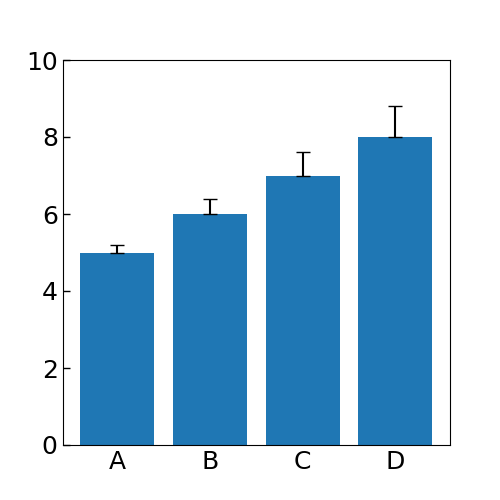# How to describe error bars directed above only

Excuse me. I want to show an error bar directed only upper side in a bar plot (like ‘‘T’’), but lower side caption is appeared like ‘‘ｴ’’ in the png file. Do you have any method for present one side of error bars?

I wrote on python3 as below.

# coding: utf-8

import numpy as np
from matplotlib import pyplot as plt

x = [‘A’, ‘B’, ‘C’, ‘D’]
y = [5, 6, 7, 8]
yerr = [[0, 0, 0, 0],[0.2, 0.4, 0.6, 0.8]]

fig, ax = plt.subplots(figsize=(5, 5))
ax.bar(x=x, height=y, yerr=yerr, capsize=5)
ax.set_ylim(0, 10)
ax.set_yticks(np.arange(0, 10+1, 2))
ax.xaxis.set_ticks_position(‘none’)
ax.yaxis.set_ticks_position(‘left’)
ax.tick_params(which=‘both’, direction=‘in’, width=1.00, length=5, labelsize=18)
plt.show()I would just cheat and set the `zorder` less than the `zorder` of the histogram bars to hide the bottom of the error bar.

1 Like

Thank you for your answer! I was able to describe an ideal bar plot.

Then, I modified that code as below.

yerr = [0.2, 0.4, 0.6, 0.8]
ax.bar(x=x, y=y, yerr=yerr, capsize=5, zorder=2, error_kw=dict(zorder=1))